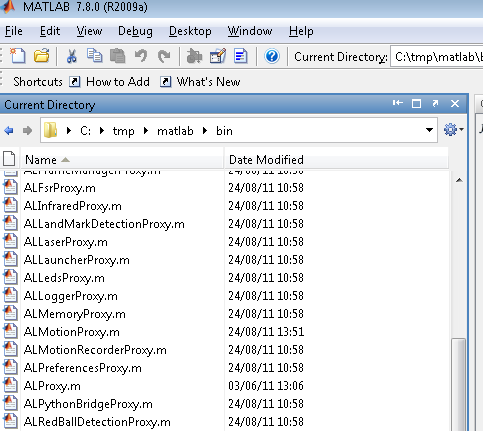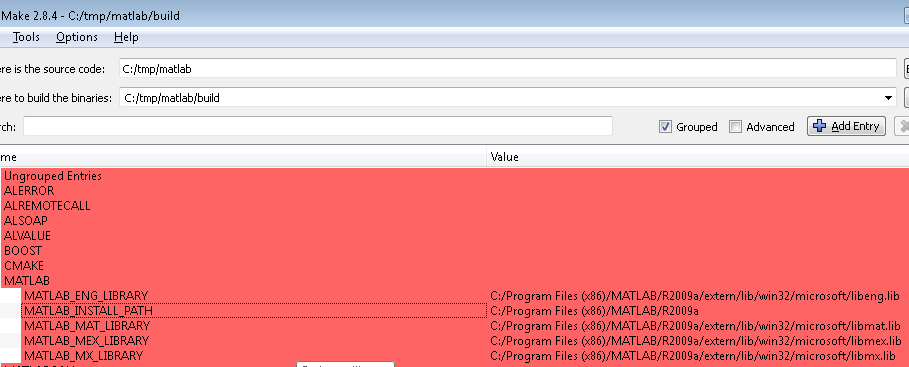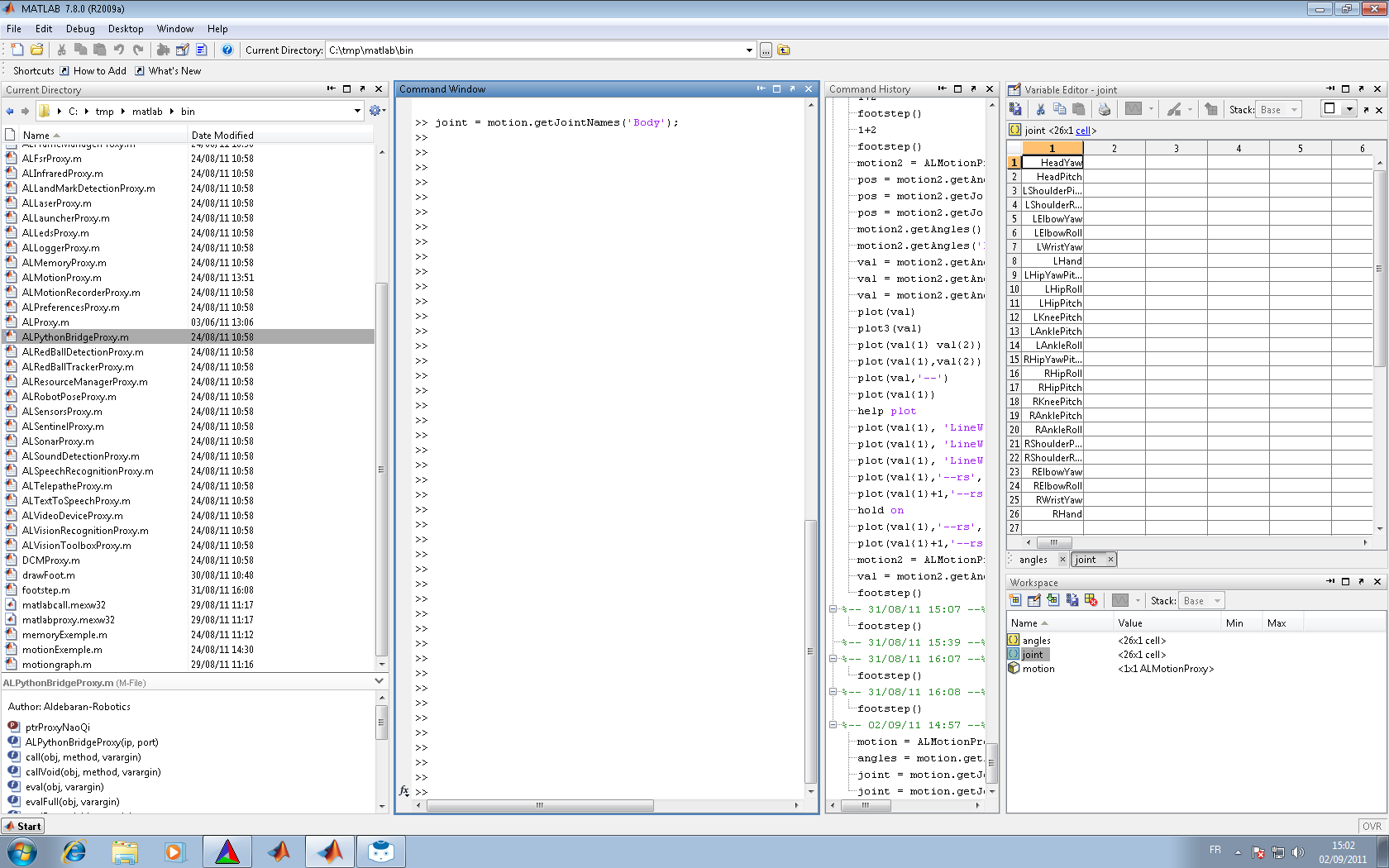# Matlab SDK¶

Overview | C++ | Python | .Net | Java | Matlab | Urbi

## Introduction¶

NaoQi-Matlab-SDK allows you to call any NaoQi module method in Matlab script. Call syntax is the same as c++, java, python or .Net. All calls are made remotely from the pc with Matlab to real or simulated robot.

Requirements:
• Matlab
• Naoqi C++ SDK (for source code compilation)
• NaoQi Matlab SDK (source code and precompiled library)
Tested on:
• Matlab 2009. Windows xp 32 bits. Others configuration require modification of the CMake configuration files.

Others OS may need some changes on CMakeLists or sources code.

## Use precompiled Matlab library¶

### Content¶

• All c++ module API converted to matlab. For example, ALMemoryProxy.m contains all ALMemory method’s. See module APIs for more information.
• Matlab proxy library
• Matlab call library
• memory example: insert a variable in ALMemory with matlab.
• footStep example: trace footstep with Matlab

### Use precompiled Matlab library¶

Point matlab to the toolbox folder with matlab interface:## Compilation¶

### Windows¶

Use CMake to compile the naoqi-Matlab-SDK.

```z\$ cd matlab
z\$ mkdir build
z\$ cd build
z\$ cmake -DCMAKE_TOOLCHAIN_FILE=sdkpath/naoqi-sdk-1.12.0.26-linux32/toolchain-pc.cmake ..```
Depending your matlab version, CMake may not find Matlab install folder. In CMake GUI or with command line, fill the matlab variable:
• \${MATLAB_INSTALL_PATH} for example C:\Program Files (x86)\MATLAB\R2009a
• \${MATLAB_LIBRARY_DIR} for example C:\Program Files (x86)\MATLAB\R2009a\extern\lib\win32\microsoft
• \${MATLAB_INCLUDE_DIR} for example C:\Program Files (x86)\MATLAB\R2009a\extern\include

and configure again.## Examples¶

### Get joint name in cells matrix¶

```motion = ALMotionProxy('127.0.0.1');
names = motion.getAngles('Body',false);
```You can see a matrix named “names” with all joints name.

### Helloworld example¶

```tts = ALTextToSpeechProxy('myRobotIP',9559);
tts.say('hello world');
```

### ALMemory::insertData example¶

```mem = ALMemoryProxy('127.0.0.1',9559);
mem.insertData('matlabVariable',5);
```

### ALMotion::getFootStep example¶

```function footstep()
'motion footstep graph'

motion = ALMotionProxy('127.0.0.1',9559);

for z = 1:20

step = motion.getFootSteps();
left = step{1}{1};
right = step{1}{2};
if( (left{1} ~= right{1}) && (left{2} ~= right{2}))
left2D = {left{1},left{2},left{3}}
right2D = {right{1},right{2},right{3}}
end
if (size(step{1})>=1)
for i = 1:size(step{2});

name = step{2}{i}{1}
pos = step{2}{i}{3}
tmpPose2D = {pos{1}, pos{2}, pos{3}};
if(name == 'LLeg')
left2D{1} = right2D{1} + cos(right2D{3}) * tmpPose2D{1} - sin(right2D{3}) * tmpPose2D{2};
left2D{2} = right2D{2} + sin(right2D{3}) * tmpPose2D{1} + cos(right2D{3}) * tmpPose2D{2};
left2D{3} = right2D{3} + tmpPose2D{3};
drawFoot(left2D{1}, left2D{2}, left2D{3}, [1 0 0], 2);
else
right2D{1} = left2D{1} + cos(left2D{3}) * tmpPose2D{1} - sin(left2D{3}) * tmpPose2D{2};
right2D{2} = left2D{2} + sin(left2D{3}) * tmpPose2D{1} + cos(left2D{3}) * tmpPose2D{2};
right2D{3} = left2D{3} + tmpPose2D{3};
drawFoot(right2D{1}, right2D{2}, right2D{3}, [0 1 0], 2);
end
end
end
if (size(step{3})>=1)
for i = 1:size(step{3});
name = step{3}{i}{1};
pos = step{3}{i}{3};
tmpPose2D = {pos{1}, pos{2}, pos{3}};
if(name == 'LLeg')
left2D{1} = right2D{1} + cos(right2D{3}) * tmpPose2D{1} - sin(right2D{3}) * tmpPose2D{2};
left2D{2} = right2D{2} + sin(right2D{3}) * tmpPose2D{1} + cos(right2D{3}) * tmpPose2D{2};
left2D{3} = right2D{3} + tmpPose2D{3};
drawFoot(left2D{1}, left2D{2}, left2D{3}, [1 0 0], 0.5);
else
right2D{1} = left2D{1} + cos(left2D{3}) * tmpPose2D{1} - sin(left2D{3}) * tmpPose2D{2};
right2D{2} = left2D{2} + sin(left2D{3}) * tmpPose2D{1} + cos(left2D{3}) * tmpPose2D{2};
right2D{3} = left2D{3} + tmpPose2D{3};
drawFoot(right2D{1}, right2D{2}, right2D{3}, [0 1 0], 0.5);
end
end
end

pause(1.0)
end
end
```

## Using Naoqi.Net with Matlab¶

On Microsoft Windows, Matlab allows you to use a .Net library from within an m file. To use this approach, please first follow the instructions for installing naoqi.net. Once installed, you can use the following approach in an matlab file.

```NET.addAssembly('C:\Program Files (x86)\Aldebaran\NAOqi.Net SDK *.*.*.*\naoqi-dotnet4.dll')
m = Aldebaran.Proxies.MotionProxy('127.0.0.1',9559)
m.getSummary()
m.getAngles('Body',true)
angles = m.getAngles('Body',true)

tts = Aldebaran.Proxies.TextToSpeechProxy('robotName.local',9559)
tts.say('Hello from Matlab through DotNet.')
```

## Type conversion¶

Module API methods sometimes require or return an ALValue. ALValue is a Cell or Cell Array in Matlab and can often be converted to a simple matlab type.

C++ Matlab
bool bool
int int32
float double
string string
ALValue::binary not managed
vector<float> cells array
vector<int> cells array
vector<string> cells array
ALValue cells